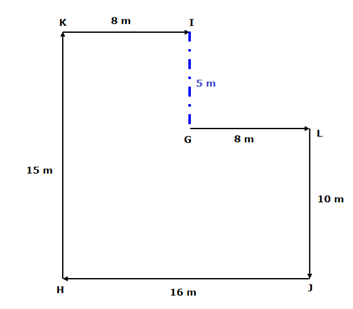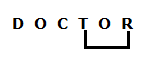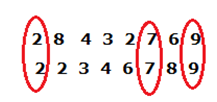# IBPS Clerk Prelims Reasoning (Day-03)

Dear Aspirants, Our IBPS Guide team is providing new series of Reasoning Questions for IBPS Clerk Prelims 2020 so the aspirants can practice it on a daily basis. These questions are framed by our skilled experts after understanding your needs thoroughly. Aspirants can practice these new series questions daily to familiarize with the exact exam pattern and make your preparation effective.

Start Quiz

Number series

Direction (1-5): Following questions are based on the five three-digit numbers given below.

124   465   321   576   865

1) If all the digits in each of the numbers are arranged in ascending order within the number, then which of the following number is the highest number?

A) 321

B) 124

C) 576

D) 865

E) 465

2) If all the numbers are arranged in descending order from left to right, then which of the following will be the product of the 3rd digit of 2nd number from the left end and 3rd digit of 2nd number from the right end?

A) 12

B) 6

C) 10

D) 15

E) 7

3) What will be the resultant when 3rd digit of the highest number is multiplied with the 2nd digit of the lowest number?

A) 16

B) 10

C) 8

D) 24

E) 20

4) If the position of the second and the third digits of each of the numbers are interchanged, then how many odd numbers will be formed?

A) 0

B) 4

C) 3

D) 1

E) 2

5) If one is subtracted from each of the numbers, then how many numbers thus formed will be divisible by 4?

A) 4

B) 3

C) 1

D) 2

E) 0

Direction sense

Direction (6-8): Study the following information carefully and answer the below questions.

Point L is 8 m to the east of point G. Point J is 10 m to the south of point L. Point H is 16 m to the west of point J. Point K is 15 m to the north of point H. The point I is 8 m to the east of point K.

6) In which direction is point L with respect of point H?

A) North-West

B) North-East

C) South-West

D) South-East

E) South

7) What is the shortest distance between G and I?

A) 12 m

B) 16 m

C) 10 m

D) 5 m

E) None of these

8) In which direction is point J with respect to point K?

A) South

B) South-East

C) North-East

D) West

E) North-West

#### Misc

9) How many pairs of letters are there in the word ‘DOCTOR’ each of which has as many letters between them in the word as they have between them in the English alphabet in forward and backward direction?

A) 2

B) 4

C) 3

D) 1

E) None

10) How many such numerals are there in the number ‘28432769’ which will remain at the same position when arranged in ascending order from left to right?

A) 2

B) 1

C) 3

D) More than three

E) None

Directions (1-5) :

The new arrangement is- 124 456 123 567 568

After the arrangement, 2ndnumber from the left end is – 576

And 2ndnumber from the right end is – 321

So, 6*1=6

3rd digit of the highest number is – 5

2nd digit of the lowest number is – 2

Their product is 10

After the arrangement, the series is – 142 456 312 567 856

After the arrangement, the series is – 123 464 320 575 864

Directions (6-8):